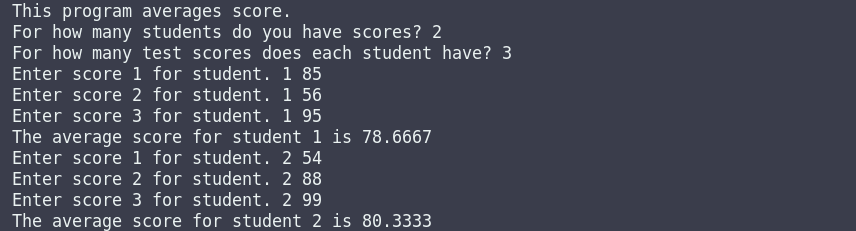Write a program that computes the average test scores of students. The program should first ask the user to input the total number of students and then the number of test scores per student. After that, for each student, ask the user to enter test scores and compute (and display) the average test score of every student, as shown in the sample output below:

Sample Input:

This program will average score.

For how many students do you have scores? 2

For how many test scores does each student have? 3

Enter score 1 for the student. 1 85

Enter score 2 for the student. 1 56

Enter score 3 for the student. 1 95

The average score for student 1 is 78.6667

Enter score 1 for the student. 2 54

Enter score 2 for the student. 2 88

Enter score 3 for the student. 2 99

The average score for student 2 is 80.3333

#include <iostream>
using namespace std;

void AverageOfStudents()
{
int numberOfStudents;
int numOfTestScores;
float testScore;
float average = 0.0;
cout<<"This program will averages score.\n";
cout<<"For how many students do you have scores? ";
cin>>numberOfStudents;
cout<<"For how many test scores does each student have? ";
cin>>numOfTestScores;
for (int i = 1; i<= numberOfStudents; i++)
{
for (int j = 1; j <= numOfTestScores; j++)
{
cout<<"Enter score "<<j<<" for student. "<<i<<" ";
cin>>testScore;
average += testScore;
}
cout<<"The average score the for student "<<i<<" is "<<average/numOfTestScores<<endl;
average = 0;
}
}
int main()
{
AverageOfStudents();

return 0;
}c++ exercises and solutions